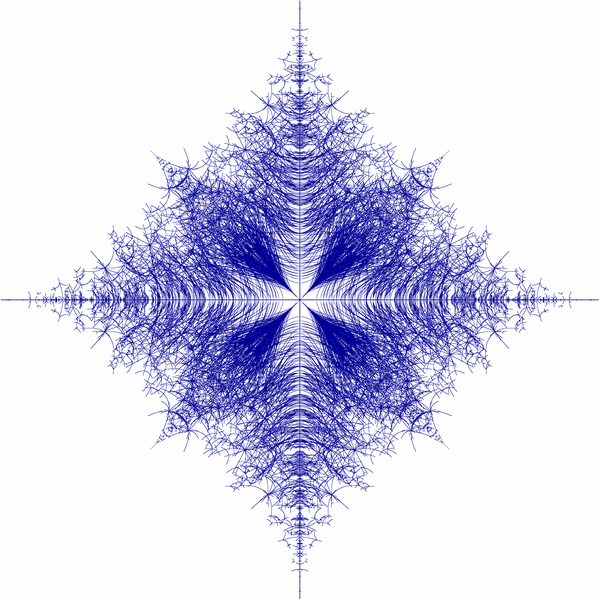TUHH / Institut für Mathematik / Forschungsgebiete / Fredholm and Spectral theory# Fredholm and Spectral theory

### Collaborators (MAT): Prof. Dr. Marko Lindner

We are interested in equations $$Ax = b$$, where $$A$$ is a linear operator acting boundedly between Banach spaces. $$A$$ is invertible (i.e. it has an inverse, $$A^1$$, that is again bounded and linear) if and only if $$A$$ is injective (its nullspace, the set of solutions $$x$$ to the homogeneous equation $$Ax = 0$$, consists of $$0$$ only) and surjective (its image/range is the whole space). Deviation from both properties, and hence from invertibility, can be measured in terms of the dimension of the nullspace and the codimension of the image. One says that $$A$$ is a Fredholm operator if both numbers are finite ? so injectivity and surjectivity might be violated but only to some (finite dimensional) extent. In particular, $$Ax = b$$ is solvable for all $$b$$ in a space of finite codimension and the solution $$x$$ is unique up to perturbations from a finite-dimensional space. The Fredholm property is robust under certain perturbations which makes it more accessible than (but still in touch with) invertibility. If you want to prove that your operator $$A$$ is invertible (i.e. $$Ax = b$$ has a unique solution $$x$$ for all b) it is often a good idea (and a big step in the right direction) to start with its Fredholm property.

Typical decay properties of infinite matrices under investigation.left: band matrices, right: norm-limits of such

Our operators $$A$$ usually appear as infinite matrices $$(A_{ij})$$ ? possibly with $$i,j \in \mathbb{Z}^d$$.

and $$A_{ij}$$ operator-valued ? usually coming from integral or differential equations (and their discretizations ). Our studies on invertibility and Fredholm property give us (in general lower) bounds on spectrum and essential spectrum of $$A$$.Figure: Spectra of non-selfadjoint Hamiltonian of randomly hopping particle in $$1D$$